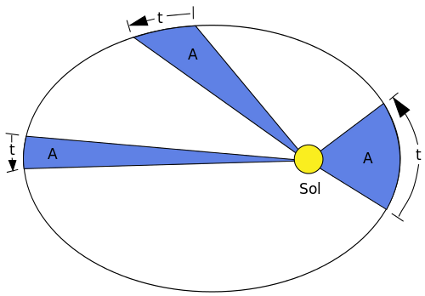# No AttractionKepler’s second law holds that a line segment connecting an orbiting planet to its sun sweeps out equal areas in equal periods of time: In the diagram above, if the time intervals t are equal, then so are the areas A.

If gravity were turned off, would this still be true?